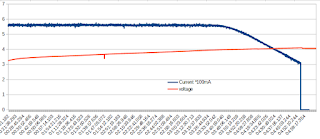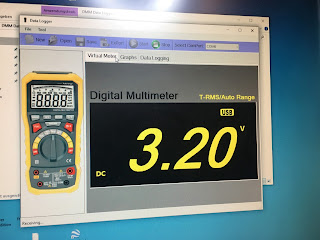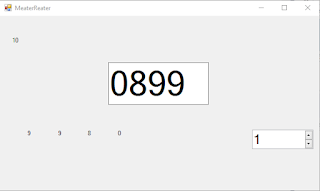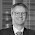## Friday, 24 February 2017

### How I got into this

When I worked on a review of a battery charger, I came accross some potential issues that I had to investigate things more thoroughly. I needed a multimeter to record the charge curves.
So my contact at Gearbest sent me this BSide ADM20 Multimeter. This has a built-in USB interface to display and record mesaurements on the PC.Values imported into LibreOffice Calc
It turned out I quite like the meter. See my review video here. (Hardware-hack will follow) The software however was rather basic and wouldn't allow to set a sample rate or measurement duration.

The meter is available under several names:

I already had a look inside the meter and see pretty cool options to turn this into an IoT device. But let's not jump to conclusions. Some more work needs to go into that and I have only focussed on the software side here.

### Plug&Play

Fortunately it is pretty obvious how the meter communicates with (or rather "to") the PC:
A new COM port appears, presented through the well known CH340 USB-to-SERIAL bridge driver.And you thought COM-Ports were a thing of the past
If you then fire up the software (DMM Data logger) that came with the meter, you're good to go.Original Software

Nooo! Boooooooring!!!!

### A look at the protocol

Pretty obvious that I should see something when I start a a terminal program like TeraTerm od Putty.
In part 2 of this post, you'll see that this is strictly a one-way communication. So we can't talk back to the meter.

• The port speed is 2400 baud.
• There is no CR or LF at the end of each data set (see below)
• The usual 8n1 seems to apply
• Continuous stream of data: no xon/xoff
• No return channel

With the width set properly, TeraTerm's hex mode shows a pattern:The 5Fs are the Zeroes, the DF has the decimal point

Whatever I do, the transmission always starts with a series of HEX values: AA5552240110
followed by four bytes that change when stuff moves on the display. I could map the values to the following displayed digits: (excerpt from my visual basic prog)

If SerVal = 95 Then measured = 0
If SerVal = 6 Then measured = 1
If SerVal = 107 Then measured = 2
If SerVal = 47 Then measured = 3
If SerVal = 54 Then measured = 4
If SerVal = 61 Then measured = 5
If SerVal = 125 Then measured = 6
If SerVal = 7 Then measured = 7
If SerVal = 127 Then measured = 8
If SerVal = 63 Then measured = 9

It turns out that the most significant bit is the decimal point, the other bits map to the seven segments. It also sends the measured unit and the polarity further back in the data stream. Up to now I choose to ignore all of that.

The four bytes with the four digits are in reverse order, of course, for more programming fun.

So my VisualBasic program listens for the "AA555224110" sequence and then decodes the four following bytes.

I suspect that the data stream is derived from the communication with the display driver, as many bits in the data stream can directly be mapped to segments on the display.

More on those details in the second part where I will look at the hardware of both the meter and it's communication.

### First try in VisualBasicNo decimal point yet. That was once a 9v battery

If you want to have a go at the experimental code, here is where I left off for the moment:

`````` Imports System.Threading.Tasks
Imports System.Timers
Imports System.IO
Imports System.IO.Ports
Public Class Form1
Dim datensatz As String
Dim rohwert As Integer
Dim werte(22) As Integer
Dim decodewerte(4) As Integer
Dim recorddata As Boolean = False
Dim i As Integer = 0
Delegate Sub DataDelegate(ByVal sdata As Integer)
REM Define the method (Function) that will be called by the Invoke method
Private Sub PrintData(ByVal sdata As Integer)
Dim startsequence As String = "AA555224110"
Dim tmpchar As String
Dim str As Integer
Dim measured As Integer
Dim x As Integer
If recorddata Then
werte(i) = sdata
Console.Write("I= ")
Console.WriteLine(i)
If i = 4 Then
recorddata = False
i = 0
tmpchar = Hex(werte(1))
REM Console.WriteLine(werte(1))
x = DecodeValue(werte(1))
decodewerte(1) = x
Console.WriteLine(x)
Label2.Text = x
tmpchar = Hex(werte(2))
REM Console.WriteLine(werte(2))
x = DecodeValue(werte(2))
decodewerte(2) = x
Console.WriteLine(x)
Label3.Text = x
tmpchar = Hex(werte(3))
REM Console.WriteLine(werte(3))
x = DecodeValue(werte(3))
decodewerte(3) = x
Console.WriteLine(x)
Label4.Text = x
tmpchar = Hex(werte(4))
REM Console.WriteLine(werte(4))
x = DecodeValue(werte(4))
decodewerte(4) = x
Console.WriteLine(x)
Label5.Text = x
TextBox1.Text = CStr(decodewerte(4)) & CStr(decodewerte(3)) & CStr(decodewerte(2)) & CStr(decodewerte(1))
End If
i = i + 1
End If
tmpchar = Hex(sdata)
Label1.Text = tmpchar
datensatz = datensatz + tmpchar
Console.WriteLine(datensatz)
If (datensatz.Contains(startsequence)) Then
datensatz = ""
recorddata = True
End If
End Sub
Public Sub New()
' This call is required by the designer.
InitializeComponent()
' Add any initialization after the InitializeComponent() call.
End Sub
Dim WithEvents sp As New SerialPort
Private Sub GetSerialPortNames()
sp.BaudRate = 2400
sp.PortName = "COM3"
sp.Open()
sp.DataBits = 8
sp.Parity = Parity.None
sp.StopBits = StopBits.One
sp.Handshake = Handshake.None
REM sp.Encoding = System.Text.Encoding.Default
sp.Encoding = System.Text.Encoding.Default
End Sub
Private Sub Form1_Load(ByVal sender As System.Object, ByVal e As System.EventArgs) Handles MyBase.Load
GetSerialPortNames()
End Sub
Dim str As Integer
REM Dim str2 As Char
REM Console.WriteLine(str)
REM str2 = Convert.ToChar(str)
End Sub
Function DecodeValue(ByVal SerVal As Integer)
Dim decimalpoint As Boolean = 0
Dim measured As Integer
If SerVal > 128 Then
SerVal = SerVal - 128
decimalpoint = True
End If
measured = 99
If SerVal = 95 Then measured = 0
If SerVal = 6 Then measured = 1
If SerVal = 107 Then measured = 2
If SerVal = 47 Then measured = 3
If SerVal = 54 Then measured = 4
If SerVal = 61 Then measured = 5
If SerVal = 125 Then measured = 6
If SerVal = 7 Then measured = 7
If SerVal = 127 Then measured = 8
If SerVal = 63 Then measured = 9
If SerVal = 8097 Then measured = 7
If SerVal = 8096 Then measured = 1
If SerVal = 0 Then measured = 0
Console.Write("Decoder got a: ")
Console.Write(SerVal)
Console.Write(" decoded as: ")
Console.WriteLine(measured)
Return measured
End Function
End Class
``````

If you have done work on hard- or software-hacking those meters please let me know.

1.There is a more sophisticated software UltraDMM for data logging and the list of supported chips: http://www.ultradmm.com/SupportedMeters.aspx
Which chip is inside of BSIDE ADM20?

1.I didn't know UltraDMM. Although it hasn't been updated for four years, it looks great.
At the moment my lab is stowed away because of building renovations, so I can't test it now. But it is certainly worth trying if UltraDMM supports the ADM20.

2.Hello. I experimented with the firmware from my adm20 and accidentally erased the original firmware. Could you post it?

2.http://www.kerrywong.com/2016/03/19/hacking-dtm0660l-based-multimeters/

3.Hi andreas, i bought (after i saw your great videos which gave me confidence!) the perfect adm20 about a year and a half ago, and now i wanted to get another one.. But i can't find listing for that on ebay etc
Do you know where i can get new one?
If not, is the mustool and peakmeter are exactly the same just branded and coloured different? ( is it same mb, chips, performance, QUALITY! And probes?)
I'M REALLY like this meter, just perfect!!!
Thanks!

4.Hi
Andreas, i bought the adm20 about a year and a half ago ( after I saw your videos which gave me confidence)
And because it's so perfect, I'm want to buy another one.. But cant find it on ebay etc Do you know where i can get an new one?
If not, is the mustool and peakmeter are exactly the same just branded and coloured different? ( is they same QUALITY, PROBES, mb, hardware,function and accurate..?

5.@Litec Systems
search for Bolyfa BF117 multimeter on Amazon.com

1.Thanks but ive got already the pm8236
Compared to my bside ;
Pros- softer buttons, maybe even too much
Cons the probes extremely less comfort than the adm20

6.Andreas,
In case you're still looking for software for the multimeter